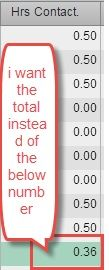## Sum of averages

Dear All ,

I have question and request help ..

I have three columns A ,B and C

A column aggregation is taken as SUM

B column aggregation is taken as Average

C colimn aggregation is taken sa SUM

Now when i choose the option of column sub totals  for B column i find the average is being displayed ...

is there any workings where we can get the total of averages for the B column ?

Regards

Soman

6 REPLIES 6

## Re: Sum of averages

wating for some to revert on my query

## Re: Sum of averages

Hi Soman,

You can try this...

Create an Aggregated Measure

Avg [_ByGroup_] ('B'n) / Avg [_ForAll_] ('B'n)

Instead of Avg you can take SUM...

Hope this will help..

Let me know

Thanks & Regards,

Teja Surapaneni

## Re: Sum of averages

HI teja ,

it did not work , i have enlosed an image for u , so that u can have clear view of the issue

request u r input

regards## Re: Sum of averages

Hello Soman,

i have seen your screen shot.

As per my knowledge it is not possible..

let's hope someone can help..

Thanks & Regards,

Teja Surapaneni.

## Re: Sum of averages

Column sub totals and totals is aggregated based on the statistic used. There isn't a way to override the statistic calculation you desire in the default cross table object.

If Averages are chosen then the sub total/total is an average. It doesn't make sense to see a sub total as the sum of averages when the underlying reported line items are averages.

Kind Regards,

Michelle

//Contact me to learn how Metacoda software can help keep your SAS platform secure - https://www.metacoda.com

## Re: Sum of averages

I don't think this is possible. You cannot set the aggregation type in the data pane for an aggregated measure, so you cannot sum averages.

Remember that VA does not use the contents of the table to determine the totals, it uses the underlying dataset. So where the averages in the table are a [_ByGroup_] average, the total shown under the table is the same aggregation but as a [_ForAll_] average.

Discussion stats
• 6 replies
• 1940 views
• 0 likes
• 4 in conversation